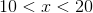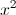## Example Questions

### Example Question #1 : How To Find The Square Of An Integer

Neither x nor y is equal to 0.

xy = 4y/x

Quantity A: x

Quantity B: 2

Quantity B is greater.

The relationship cannot be determined from the information given.

Quantity A is greater.

The two quantities are equal.

The relationship cannot be determined from the information given.

Explanation:

Given xy = 4y/x and x and y not 0.

Therefore you are able to divide both sides by 'y' such that:

x = 4/x

Multiply both sides by x:

x2 = 4 or x = +2 or –2.

Because of the fact that x could equal –2, the relationship cannot be determined from the information given.

### Example Question #1 : ArithmeticQuantity A:Quantity B: 399

The two quantities are equal.

The relationship cannot be determined from the information given.

Quantity A is greater.

Quantitiy B is greater.

The relationship cannot be determined from the information given.

Explanation:

Sinceis between 10 and 20, it can be any real number between 100 and 400. Therefore, the relationship cannot be determined sincecould fall anywhere between these two limits, including between 399 and 400.

### Example Question #1 : Arithmetic

Quantity A: 9

Quantity B: √(25 + 55)

Quantity A is greater.

The two quantities are equal.

Quantity B is greater.

The relationship cannot be determined from the information given.